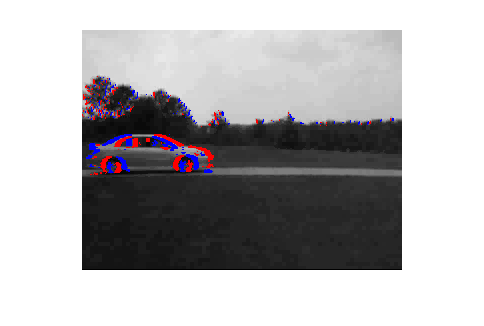# bp1

finding differences in images

## Contents

```% original images
subplot(2,1,1);
imshow(m1);
subplot(2,1,2);
imshow(m2);
disp('image size');
disp(size(m1));
```
```image size
240   320     3

```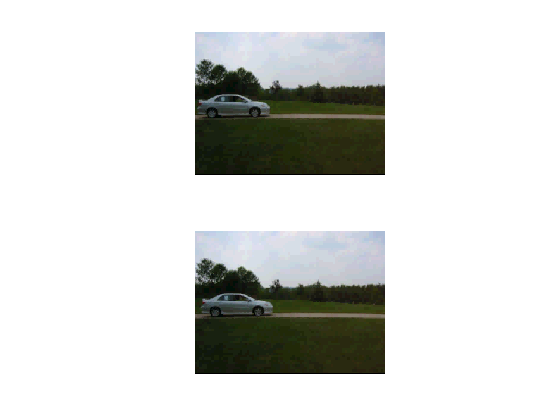## convert to grayscale and find differences

```close all
g1 = im2double(rgb2gray(m1));
g2 = im2double(rgb2gray(m2));
d = g2 - g1;
dmax = max(max(d));
dmin = min(min(d));
fprintf('max diff  %g\nmin diff %g\n',dmax,dmin);
```
```max diff  0.556863
min diff -0.509804
```

## show differences

One way of illustrating differences is to map the image range -1 .. 1 into 0 .. 1 by (1+diff)/2

```cdiff = (1+d)/2;
imshow(cdiff);
```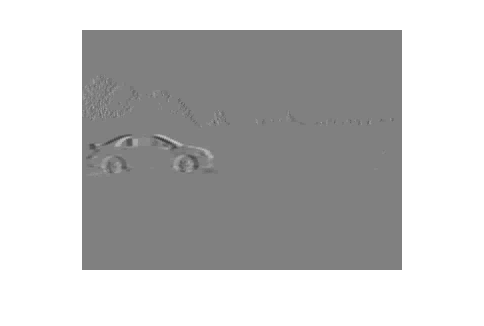## difference histogram

```% zero difference corresponds to 0.5 brightness
imhist(cdiff);
```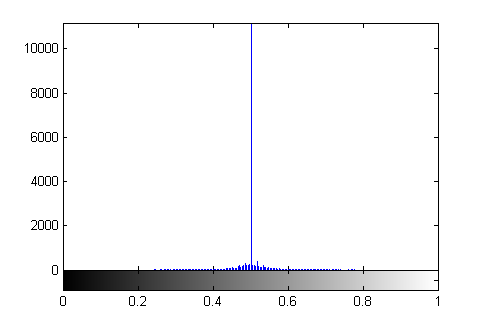```% alternative plot
[counts,x] = imhist(cdiff);
counts(129)=0; % don't count zero differences
stem(x-0.5,counts,'.');
```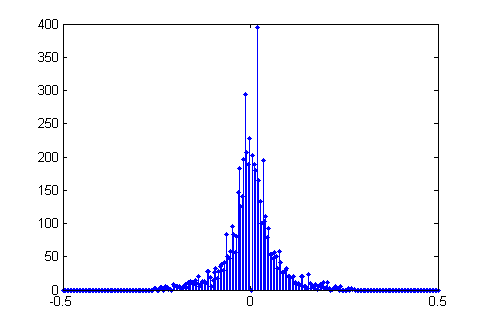## color difference display

red is positive difference, blue is negative difference

```% black background
bipolar_image(d,1);
```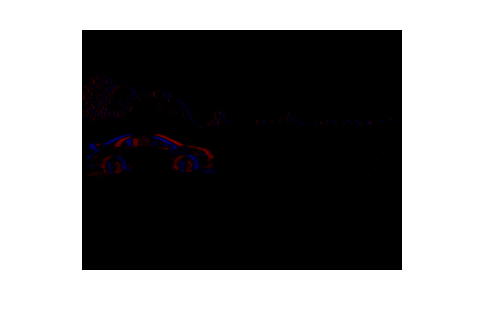```% white background
bipolar_image(d,2);
``````% superimpose on image
thres = 0.15*max(dmax,-dmin);
k1 = d < -thres;
k2 = d >  thres;
g = (g1.*not(k1)).*not(k2);
final = cat(3,g+double(k2),g,g+double(k1));
imshow(final);
```